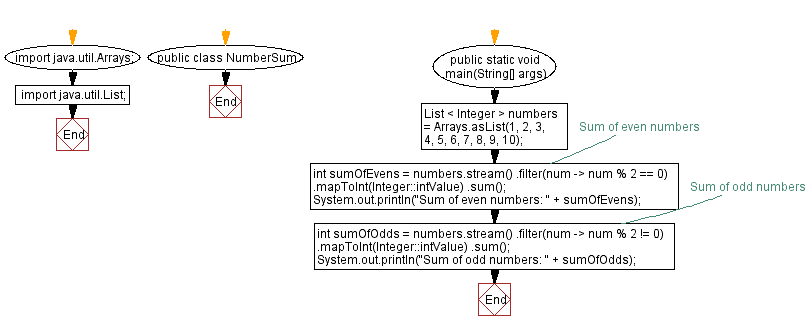﻿ Java Program: Sum of Even and Odd Numbers in a List using Streams

# Java Program: Sum of Even and Odd Numbers in a List using Streams

## Java Stream: Exercise-3 with Solution

Write a Java program to calculate the sum of all even, odd numbers in a list using streams.

Sample Solution:

Java Code:

``````import java.util.Arrays;
import java.util.List;

public class NumberSum {
public static void main(String[] args) {
List < Integer > numbers = Arrays.asList(1, 2, 3, 4, 5, 6, 7, 8, 9, 10);

// Sum of even numbers
int sumOfEvens = numbers.stream()
.filter(num -> num % 2 == 0)
.mapToInt(Integer::intValue)
.sum();

System.out.println("Sum of even numbers: " + sumOfEvens);

// Sum of odd numbers
int sumOfOdds = numbers.stream()
.filter(num -> num % 2 != 0)
.mapToInt(Integer::intValue)
.sum();

System.out.println("Sum of odd numbers: " + sumOfOdds);
}
}
```
```

Sample Output:

```List of numbers: [1, 2, 3, 4, 5, 6, 7, 8, 9, 10, 12, 14]

Sum of even numbers: 56
Sum of odd numbers: 25
```

Explanation:

In the above exercise, we have a list of integers [1, 2, 3, 4, 5, 6, 7, 8, 9, 10]. Using streams, we can filter the even numbers by applying a filter with the condition num % 2 == 0, and then sum them using the sum method. Similarly, filter odd numbers by applying a filter with the condition num % 2 != 0, and then sum them. Finally, the sums of even and odd numbers are printed to the console.

Flowchart:Java Code Editor:

Improve this sample solution and post your code through Disqus

What is the difficulty level of this exercise?

Test your Programming skills with w3resource's quiz.

﻿